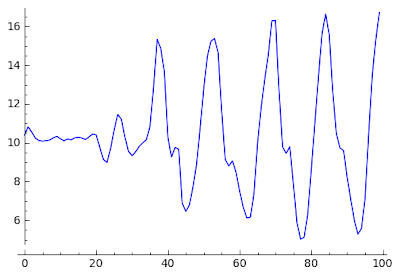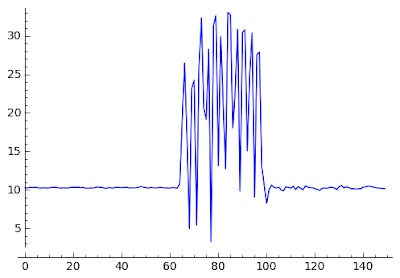# My life with Android :-)

## Friday, May 14, 2010

### Analysing acceleration sensor data with wavelets

Before we get back to Android programming, we need some theoretical background on signal analysis.The document is somewhat heavy on math. To quote Dr. Kaufman's Fortran Coloring Book: if you don't like it, skip it. But if your teacher likes it, you failed.

If you are too impatient to read, here is the essence.

There is no single perfect algorithm when analysing acceleration signals. The analysis framework should provide a toolbox of different algorithms, some working in the time-domain, some operating in the frequency domain. The decision engine that classifies the movements may use a number of algorithms, a characteristic set for each movement type.

It has been concluded in the medical research community that wavelet transformation is the most optimal algorithm for frequency-domain analysis of acceleration signals. This report presented concrete cases, how wavelet transformation can be used to classify three common movements: walking, running and shake. In addition, the wavelet transformation provided data series that can be used to extract other interesting information, e.g. step count.

For those who would like to repeat my experiments, I uploaded the prototype. First
you need Sage (I used version 4.3.3). Download and unpack the prototype package and enter the proto directory. Then launch Sage (with the "sage" command) and issue the following commands:

import accel
accel.movements(5)

now you will be able to look at the different waveforms, e.g.

list_plot(accel.shake_w5)

Sage is scriptable in Python. If you know Python, you will find everything familiar, if not - bad luck, you won't get far in Sage.

## Wednesday, May 5, 2010

### Movement patterns

This blog entry does not have associated example program, the samples of this post were taken using the sensor monitoring application presented here. This time I will give a taste what can be achieved by using the acceleration sensor for identifying movement patterns. Previously we have seen that the acceleration sensor is pretty handy at measuring the orientation of the device relative to the Earth's gravity. At that discussion we were faced with the problem what if the device is subject to acceleration other than the Earth's gravity. In that measurement, it caused noise. For example if you move the device swiftly, you can force change between landscape and portrait mode even if the device's relative position to the Earth's surface is constant. That's because the acceleration of the device's movement is added to the Earth's gravity acceleration, distorting the acceleration vector.

In this post, we will be less concerned about the device's orientation. We will focus on these added accelerations. Everyday movements have characteristic patterns and by analysing the samples produced by the acceleration sensor, cool context information can be extracted from the acceleration data.

The acceleration sensor measures the acceleration along three axes. This 3D vector is handy when one wants to measure orientation of e.g. the gravity acceleration vector. When identifying movements, it is more useful to work with the absolute value of the acceleration because the device may change its orientation during the movement. Therefore we calculate

ampl=sqrt( x^2 + y^2 + z^2 )

where x,y,z are elements of the measured acceleration vector along the three axes. Our diagrams will show this ampl value.This is actually me walking at Waterloo station. The diagram shows the beginning of the walking. It starts with stationary position then I start to walk. In order to understand the pattern, we must remember that acceleration is change of the velocity vector. This means that if we move quickly but our velocity does not change, we have 0 acceleration. On the other hand, if we move with constant speed but our direction changes, that's quite a significant change in the velocity vector. That is the source of the gravity acceleration, the Earth's surface (and we along with it) rotates with constant speed but as the speed is tangent to the Earth's surface, its direction constantly changes as the Earth rotates.

The same goes for walking. Whenever the foot hits the ground, its velocity vector changes (moved down, starts to move up). Similarly, when the foot reaches the highest point, it moved up and then it starts to move down. The maximums and the minimums of the acceleration amplitude are at these points. As these accelerations are added to the Earth's acceleration (9.81 m/s^2), you will see an offset of about 10. The sine wave-like oscillation of the acceleration vector's absolute value is unmistakable. The signal is not exactly sine wave but the upper harmonics attenuate quickly. You can see that my walking caused about 1.2g fluctuation (-0.6 g to 0.6 g).

Running is very similar except that the maximum acceleration is much higher - about 2 g peak-to peak.And now, everybody's favourite, the shaking. Shaking is very easy to identify. First, the peak acceleration is very high (about 3 g in our case). Also, the peaks are spike-like (very high upper harmonic content).In the following, I will present some algorithms that produce handy values when identifying these movements.